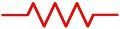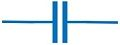# Difference Between Resistor and Capacitor

Both resistor and capacitor are passive components that are employed in electrical and electronic circuits. However, the crucial difference between the resistor and the capacitor is that a resistor is an element that dissipates electric charge or energy. As against, a capacitor is an element that stores electric charge or energy.

Basically, a resistor is used to limit the flow of current through a circuit. However, the operation of the capacitor is somewhat different from that of the resistor as it generally stores the charge and releases the stored charge whenever needed.

You will get to know about other important differences between the two. But before that look at the contents to be discussed under this article.

## Content: Resistor Vs Capacitor

### Comparison Chart

ParameterResistorCapacitor
DefinitionIt is used to restrict the flow of charge carriers in a circuit.It is used to store charges in a circuit.
SymbolUnitThe resistance of resistor is measured in ohms.The capacitance of a capacitor is measured in farads.
EquationR = V/IC = Q/V
UsesIn precision circuits, logic circuits, RF circuits etc.In waveform generation, filtering, blocking and bypass applications.

### Definition of Resistor

A resistor is the basic component of electrical and electronic circuits. It obstructs or blocks the flow of electric current flowing through the circuit. Basically, the resistance of the resistor is the energy dissipated by the resistor when the current flows through it.

Its unit is ohms and its symbol is Ω.

The figure below shows the symbolic representation of a resistor:It is majorly used to limit the charging rate of the capacitor; it also helps to adjust the frequency response of RF circuits. Also, the series combination of resistors acts as a voltage divider for the circuit.

Now, the question arises how it works:

When a resistor is connected in a circuit, then it absorbs the electrical energy that it dissipates later in the form of heat. In this way, a resistor controls the flow of charge.

All resistors exhibit 2 specific characteristics; one is its resistance which is measured in ohms and other is power dissipating capacity which is measured in watts.

Consider a resistor R, and I is the current that is flowing through that resistor. Also, V denotes the voltage drop at that particular resistor.

Then, according to ohms’ law:

V = I. R

R = V/I

On the basis of the operating conditions possessed by a resistor. It is classified into two categories:

Fixed resistor: In fixed type resistor the value of the resistance offered by the resistor to the circuit is fixed and is unchangeable.

Variable (adjustable) resistor: Variable resistors are those resistors that offer adjustable resistance value while being connected in any circuit.

### Definition of Capacitor

A capacitor is also a basic electronic component that has the ability to store an electric charge. It basically does so by making use of externally applied voltage that appears across it.

Here, the figure shows the symbolic representation of a capacitor:When a potential is provided then an electric field is generated across the capacitor. So, when charges flow through a capacitor then this generated field stores these charges.

It is to be noted here that, it basically stores the electrons thereby storing energy and emits the charges later whenever required.

A capacitor can be considered of 2 conducting plates that are held apart by a dielectric medium. Also, the dielectric material must be a poor electrical conductor or insulator.

This is shown in the figure below:When dc potential is provided across the capacitor. Then the positive and negative charges separately get accumulated at the two sides of the conducting plates.

Due to these deposited charges, the plates become neutrally charged and once the stable state is reached no current is allowed to flow through it. Hence charge is stored in it.

The capacitance of the capacitor is given as:

C = Q/V

Like a resistor, a capacitor is also classified into two categories:

Fixed capacitor: Fixed capacitor are those whose capacitance shows fixed value and not does show adjustable behaviour during circuit operation.

Variable capacitor: Like resistors, the capacitance of capacitors also shows adjustable behaviour while connected in any circuit.

## Key Differences Between Resistor and Capacitor

1. A resistor is a component that basically opposes the flow of current through the circuit in order to maintain proper voltage or the current through it. On the contrary, a capacitor is a component that stores the charges or energy in the electric field generated by the externally applied potential.
2. Resistance is the measure of the amount of energy dissipated by the resistor. While capacitance is basically but the amount of charge stored by the capacitor.
3. The resistance of the resistor is given by R = V/I. Whereas, the capacitance of the capacitor is given as C = Q/V.
4. The unit of resistance of a resistor is ohms. As against, the unit of capacitance of a capacitor is farads.
5. Resistors are majorly used in precision circuits, radio frequency and logic circuits etc. Whereas, capacitors are used in waveform generation, filtering, blocking and bypass applications.

### Conclusion

So, from the above discussion, we can conclude that both resistor and capacitor are passive components but the two acts differently when employed in any electrical or electronic circuit.